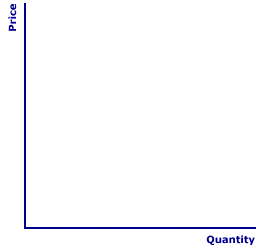Tuesday  January 31, 2023
 AmosWEB means Economics with a Touch of Whimsy!PPF: The abbreviation for production possibilities frontier, which is a curve that illustrates the production possibilities for the economy. A production possibilities frontier represents the boundary or frontier of the economy's production capabilities. That's why it's termed a production possibilities frontier (or PPF). As a frontier, it is the maximum production possible given existing (fixed) resources and technology. Producing on the curve means resources are fully employed, while producing inside the curve means resources are unemployed. The law of increasing opportunity cost is what gives the curve its distinctive convex shape.UNIT ELASTIC:

An elasticity alternative in which changes in one variable (usually price) cause equal proportional changes in another variable (usually quantity). In other words, any change in price, whether big or small, triggers exactly the same percentage change in quantity. Quantity changes match price changes. This characterization of elasticity is most important for the price elasticity of demand and the price elasticity of supply. Unit elastic is one of five elasticity alternatives. The other four are perfectly elastic, perfectly inelastic, relatively elastic, and relatively inelastic.
AlternativeCoefficient (E)
Perfectly ElasticE = ∞
Relatively Elastic1 < E < ∞
Unit ElasticE = 1
Relatively Inelastic0 < E < 1
Perfectly InelasticE = 0
Unit elastic means that any change in price causes an equal proportion change in quantity. Quantity changes are matched by price changes. More specifically, the percentage change in quantity is equal to the percentage change in price. Unit elastic demand occurs when buyers can choose from among a modest number of substitutes in the consumption of a good. In an analogous way, unit elastic supply occurs when sellers can choose among a modest number of substitutes in the production.

The chart to the right displays the five alternatives based on the coefficient of elasticity (E). In technical shorthand (which can be used by anyone with math symbols tattooed on their hands), the coefficient of elasticity (E) is given as:

E = 1

This technical shorthand works for both the price elasticity of demand and the price elasticity of supply, because the negative value of the price elasticity of demand is ignored. If the negative sign is not ignored, the price elasticity of demand is given by E = -1

### Two Curves

Unit Elastic CurvesUnit elastic demand and supply are best understood and more easily seen with pictures. The blank graph presented here is ready and willing to display a unit elastic demand curve and a unit elastic supply curve. All this is needed is click the corresponding buttons labeled [Demand] and [Supply].

Notice that the unit elastic demand curve is, in fact, a curve (rather than a straight line), while the unit elastic supply curve is a straight line that originates from the origin. Both curves are configured such that a given percentage change in price is matched by an equal percentage change in quantity. The key for demand is that the slope of the curve is steep for high prices and small quantities and flat for low prices and large quantities. The key for supply is that the line goes through the origin. If the price is zero, so too is the quantity.

### Examples Anyone?

While it would be useful to discuss a few examples of unit elastic demand and supply, such is not really possible. This is not due to any moral, religious, or philosophical objection to doing so. It is because that, unlike other elasticity alternatives, there is nothing particularly notable about goods that are unit elastic. Rather than a distinctive category, unit elastic is primarily a dividing line, a boundary, between elastic and inelastic. If the coefficient of elasticity is greater than one, then a good is elastic. If the coefficient of elasticity is less than one, then a good is inelastic. If the coefficient just happens to be exactly equal to one, then it is unit elastic. There is nothing intrinsic about a good in terms of either production or consumption that give rise to unit elastic.

 <= UNILATERAL TRANSFERS UNIT OF ACCOUNT =>Recommended Citation:

UNIT ELASTIC, AmosWEB Encyclonomic WEB*pedia, http://www.AmosWEB.com, AmosWEB LLC, 2000-2023. [Accessed: January 31, 2023].

Check Out These Related Terms...

Or For A Little Background...

And For Further Study...
Search Again?GRAY SKITTERY[What's This?] Today, you are likely to spend a great deal of time flipping through the yellow pages hoping to buy either a T-shirt commemorating last Friday (you know why) or a rotisserie oven that can also toast bread. Be on the lookout for broken fingernail clippers.Your Complete ScopeIn the Middle Ages, pepper was used for bartering, and it was often more valuable and stable in value than gold."To understand a man, you must know his memories. The same is true of a nation."-- Anthony Quayle, ActorMUMarginal UtilityA PEDestrian's Guide Xtra CreditTell us what you think about AmosWEB. Like what you see? Have suggestions for improvements? Let us know. Click the User Feedback link.| | | | | | | | | | |
| | | |

Thanks for visiting AmosWEB